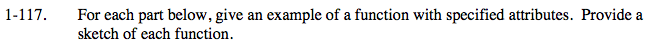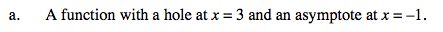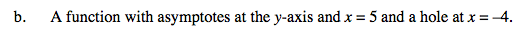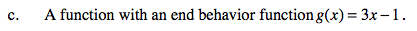### Home > CALC > Chapter 1 > Lesson 1.3.2 > Problem1-117

1-117.Write a rational function with (x − 3) in both the numerator and the denominator to make a hole at x = 3.

Remember that vertical asymptotes are formed by zeros in the denominator.An asymptote at the x axis is the same as having a vertical asymptote of x = 0.Holes and vertical asymptotes do not affect end behavior. How can y = 3x − 1 be altered so that it has a hole or vertical asymptote?

Possible answer: y = 3x − 1 + [answer to part (a)]